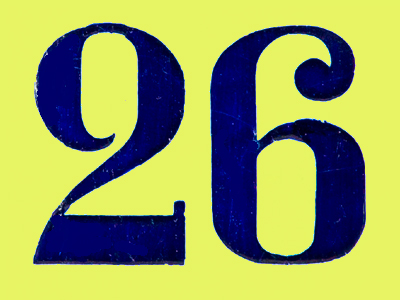26 is the next number of the sequence 5 6 8 11 15 20.

# Number Sequences 4

This Math quiz is called 'Number Sequences 4' and it has been written by teachers to help you if you are studying the subject at elementary school. Playing educational quizzes is an enjoyable way to learn if you are in the 3rd, 4th or 5th grade - aged 8 to 11.

It costs only \$12.50 per month to play this quiz and over 3,500 others that help you with your school work. You can subscribe on the page at Join Us

Number sequences are lists of numbers that follow patterns. For example, triangle numbers increase by adding 1 then 2 then 3 etc... and square numbers increase by adding 3 then 5 then 7 etc... Learning to spot the patterns enables you to know which numbers are going to come next in any given sequence.

1.
Can you find the rule of this sequence? 21, 42, 63, 84
The numbers increase in 11s
The numbers increase in 19s
The numbers increase in 21s
The numbers increase in 25s
This sequence is the 21 times table
2.
Can you find the rule of this sequence? 30, 45, 60, 75, 90
The numbers increase in 11s
The numbers increase in 15s
The numbers increase in 19s
The numbers increase in 21s
Each number (except the first) is 15 more than the ne before it
3.
What is the next number of the sequence? 12, 9, 6, 3, 0
-3
3
0.3
-6
The numbers are decreasing in 3s
4.
What is the next number of the sequence? 0.5, 1, 1.5, 2
1
2.5
3
3.5
The numbers are increasing by 0.5 each time
5.
What is the next number of the sequence? 1, 3, 6, 10, 15, 21
26
27
28
30
Number 2 is added, then 3, then 4, then 5 and so on
6.
What do we call the numbers in the sequence in question 5?
Multiples
Square numbers
Prime numbers
Triangular numbers
The sequence of triangular numbers goes up much more quickly than an ordinary sequence. Square numbers go up even more quickly
7.
The difference between two even numbers is what?
Sometimes odd and sometimes even
Always odd
Always even
It always ends in 0
Even - even = even
8.
The product of two even numbers is what?
Sometimes odd and sometimes even
Always odd
Always even
It always ends in 0
A product is the result of multiplying two or more numbers
9.
The product of two odd numbers is what?
Sometimes odd and sometimes even
Always odd
Always even
It always ends in 0
The product of two evens is always even and the product of two odds is always odd
10.
The product of an even number and an odd number is what?
Sometimes odd and sometimes even
Always odd
Always even
It always ends in 0
Even x even = even
Even x odd = even
Odd x even = even
Odd x odd = odd
Author:  Amanda Swift